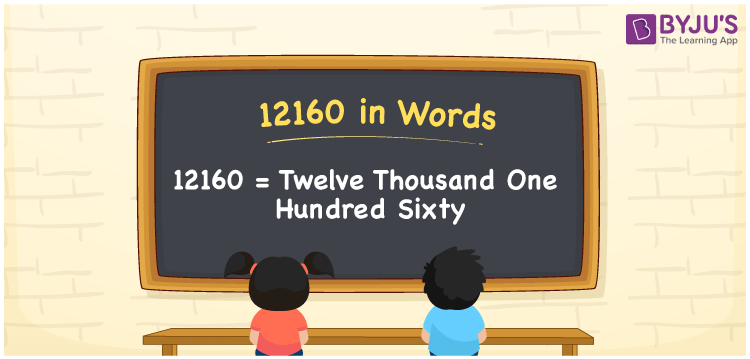# 12160 in Words

12160 in words is Twelve Thousand One Hundred Sixty. For example, if you saved Rs. 12160 in a month, you can write, “I have saved Rs. Twelve Thousand One Hundred Sixty in a month”. In general, the number name of any number can be written using the ones, tens, hundreds, and thousands place of a number. Thus, the place value chart is useful for writing the number 12160 in words.

 12160 in words Twelve Thousand One Hundred Sixty Twelve Thousand One Hundred Sixty in Numbers 12160

## 12160 in English Words## How to Write 12160 in Words?

We can convert 12160 to words using a place value chart. This can be done as follows. The number 12160 has 5 digits, so let’s make a chart that shows the place value up to 5 digits.

 Ten thousands Thousands Hundreds Tens Ones 1 2 1 6 0

Thus, we can write the expanded form as:

1 × Ten thousand + 2 × Thousand + 1 × Hundred + 6 × Ten + 0 × One

= 1 × 10000 + 2 × 1000 + 1 × 100 + 6 × 10 + 0 × 1

= 12160

= Twelve Thousand One Hundred Sixty

12160 is the natural number that is succeeded by 12159 and preceded by 12161.

12160 in words – Twelve Thousand One Hundred Sixty

Is 12160 an odd number? – No.

Is 12160 an even number? – Yes

Is 12160 a perfect square number? – No

Is 12160 a perfect cube number? – No

Is 12160 a prime number? – No

Is 12160 a composite number? – Yes

## Frequently Asked Questions on 12160 in Words

Q1

### How to write 12160 in words?

12160 in English is written as “Twelve Thousand One Hundred Sixty”.
Q2

### Is the number 12160 an odd number?

No, the number 12160 is not an odd number.
Q3

### Is 12160 a composite number?

Yes, 12160 is a composite number.